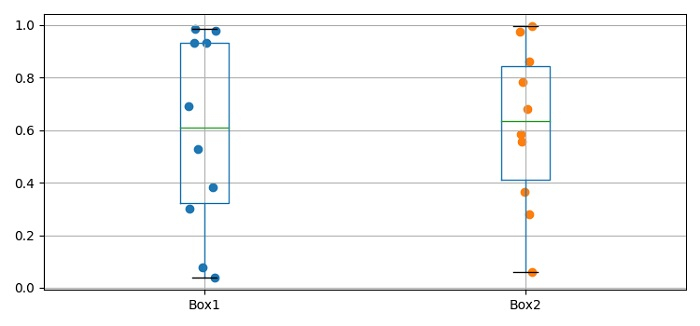# Adding a scatter of points to a boxplot using Matplotlib

MatplotlibPythonData Visualization

To add a scatter of points to a boxplot using matplotlib, we can use boxplot() method and enumerate the Pandas dataframe to get the x and y data points to plot the scatter points.

## Steps

• Set the figure size and adjust the padding between and around the subplots.

• Make a dataframe using DataFrame class with the keys, Box1 and Box2.

• Make boxplots from the dataframe.

• Find x and y for the scatter plot using data (Step 1).

• To display the figure, use show() method.

## Example

import pandas as pd
import numpy as np
from matplotlib import pyplot as plt
plt.rcParams["figure.figsize"] = [7.50, 3.50]
plt.rcParams["figure.autolayout"] = True
data = pd.DataFrame({"Box1": np.random.rand(10), "Box2": np.random.rand(10)})
data.boxplot()
for i, d in enumerate(data):
y = data[d]
x = np.random.normal(i + 1, 0.04, len(y))
plt.scatter(x, y)
plt.show()

## Output